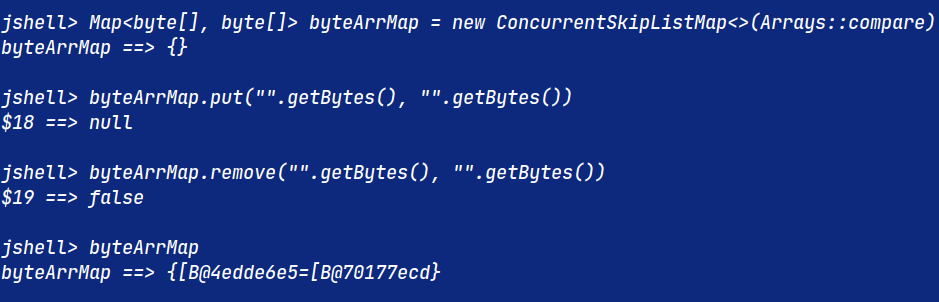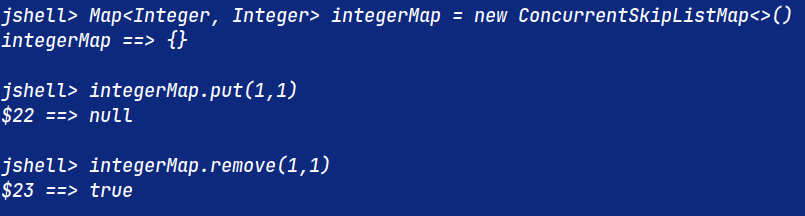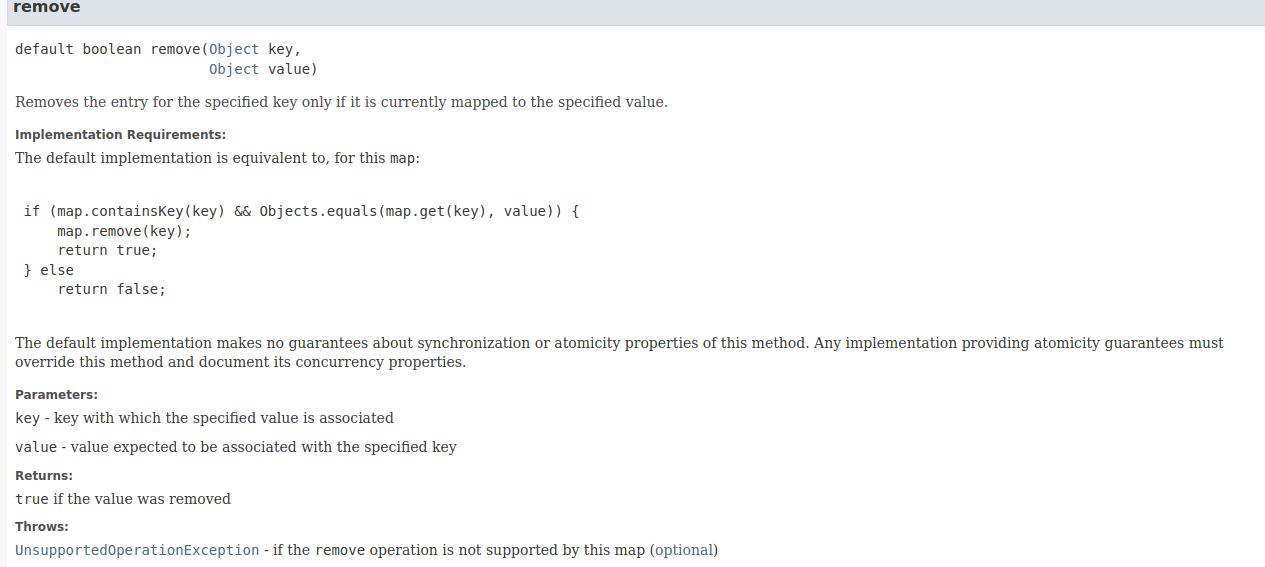# Why is it that byteArrMap.remove is returning false

## Question from theuntamed000#1481

noob question why is that byteArrMap.remove returning falsealso does java use something like Integer.valueOf() while doing boxing

aight so first one

when you call remove with a byte array those byte arrays are not equal

``````jshell> byte[] b1 = new byte;
b1 ==> byte {  }

jshell> byte[] b2 = new byte;
b2 ==> byte {  }

jshell> b1 == b2
\$3 ==> false

jshell> b1.equals(b2)
\$4 ==> false``````

even though they have the same contents

just read the docs , it uses Object.equals which uses references

So it won't find a matching key, thus it won't remove anything returning false.

Second, `new Integer(123)` and `Integer.valueOf(123`) might seem identical, but `new Integer` is a constructor call and all constructor calls need to return distinct objects.

Static methods don't have that restriction, so you can implement some degree of caching behind them. Which, looking at the implementation of `Integer.valueOf` is what is being done. Small numbers' Integer representations are cached.

``````    @IntrinsicCandidate
public static Integer valueOf(int i) {
return i >= -128 && i <= Integer.IntegerCache.high ? Integer.IntegerCache.cache[i + 128] : new Integer(i);
}``````

But the exact strategy matters less than the fact that choosing to implement a strategy is possible with the "static factory."

Even if the implementation was just

``````    @IntrinsicCandidate
public static Integer valueOf(int i) {
return new Integer(i);
}``````

there would be a value in it from a library design standpoint.

And that is why this deprecated for removal makes sense. Removing the ability for libraries to call the constructor directly means the jdk would simply have more options for optimizations.

``````    @Deprecated(
since = "9",
forRemoval = true
)
public Integer(int value) {
this.value = value;
}``````

And when the language does autoboxing - yes it uses valueOf

``````\$ cat Box.java
class Box {
Integer f() {
Integer i = 4;
return i;
}
}

\$ javac Box.java

\$ javap -v Box.class``````
``````Classfile /Users/emccue/Development/micro-http-ring/Box.class
SHA-256 checksum 78f01c27cb6b16a51a1c0ac47bf1ceb94cc5a4a7672afc615eb634dd948ba138
Compiled from "Box.java"
class Box
minor version: 0
major version: 61
flags: (0x0020) ACC_SUPER
this_class: #13                         // Box
super_class: #2                         // java/lang/Object
interfaces: 0, fields: 0, methods: 2, attributes: 1
Constant pool:
#1 = Methodref          #2.#3          // java/lang/Object."<init>":()V
#2 = Class              #4             // java/lang/Object
#3 = NameAndType        #5:#6          // "<init>":()V
#4 = Utf8               java/lang/Object
#5 = Utf8               <init>
#6 = Utf8               ()V
#7 = Methodref          #8.#9          // java/lang/Integer.valueOf:(I)Ljava/lang/Integer;
#8 = Class              #10            // java/lang/Integer
#9 = NameAndType        #11:#12        // valueOf:(I)Ljava/lang/Integer;
#10 = Utf8               java/lang/Integer
#11 = Utf8               valueOf
#12 = Utf8               (I)Ljava/lang/Integer;
#13 = Class              #14            // Box
#14 = Utf8               Box
#15 = Utf8               Code
#16 = Utf8               LineNumberTable
#17 = Utf8               f
#18 = Utf8               ()Ljava/lang/Integer;
#19 = Utf8               SourceFile
#20 = Utf8               Box.java
{
Box();
descriptor: ()V
flags: (0x0000)
Code:
stack=1, locals=1, args_size=1
1: invokespecial #1                  // Method java/lang/Object."<init>":()V
4: return
LineNumberTable:
line 1: 0
java.lang.Integer f();
descriptor: ()Ljava/lang/Integer;
flags: (0x0000)
Code:
stack=1, locals=2, args_size=1
0: iconst_4
1: invokestatic  #7                  // Method java/lang/Integer.valueOf:(I)Ljava/lang/Integer;
4: astore_1
6: areturn
LineNumberTable:
line 3: 0
line 4: 5
}
SourceFile: "Box.java"``````

a bit verbose, but you see the `invokestatic` call in the last snippet refers to `Integer.valueOf`

yeah

``````\$ javap -c Box.class
Compiled from "Box.java"
class Box {
Box();
Code:
1: invokespecial #1                  // Method java/lang/Object."<init>":()V
4: return

java.lang.Integer f();
Code:
0: iconst_4
1: invokestatic  #7                  // Method java/lang/Integer.valueOf:(I)Ljava/lang/Integer;
4: astore_1
6: areturn
}``````

easier to see with `-c` (still learning javap)

can there be case where the new Integer(i) is called

yes, if the integer is outside of the range -128 to 128 it will be outside of the cache and new Integer will be used.

for this implementation

if it can then Objects.equals() will return false , and it would behave like the byte[] example

but i guess that never happens

not quite

the implementation of equals for Integer actually compares the value

``````jshell> Integer i1 = Integer.valueOf(123456);
i1 ==> 123456

jshell> Integer i2 = Integer.valueOf(123456);
i2 ==> 123456

jshell> i1 == i2
\$7 ==> false

jshell> i1.equals(i2)
\$8 ==> true``````
``````jshell> byte[] b1 = new byte;
b1 ==> byte {  }

jshell> byte[] b2 = new byte;
b2 ==> byte {  }

jshell> b1 == b2
\$3 ==> false

jshell> b1.equals(b2)
\$4 ==> false``````

reference equality `i1 == i2` will return false if you have distinct `Integer` objectsBut the `equals` method for `Integer` inherited from `Object` is overridden so that comparing them with `.equals` or `java.util.Objects.equals(` will give the answer you would usually expect.

Yep so if you tried the `byte[]` map example with `Integer` then `.remove `would always find its target value and return `true` even if you used the deprecated `new Integer` directly or were outside of the cache range for `Integer.valueOf`.

``````BYTE ARRAYS
| b1 == b2 | b1.equals(b2) | Objects.equals(b1, b2) | Arrays.equals(b1, b2)
----------------|--------------------------------------------------------------------------
| SAME OBJECT   | true     | true          | true                   | true
----------------|--------------------------------------------------------------------------
| SAME CONTENTS | false    | false         | false                  | true
----------------|--------------------------------------------------------------------------
| DIFF CONTENTS | false    | false         | false                  | false
----------------|--------------------------------------------------------------------------
| b1 is null    | false    | crash         | false                  | false
----------------|--------------------------------------------------------------------------
| b2 is null    | false    | false         | false                  | false
----------------|--------------------------------------------------------------------------
| both are null | true     | crash         | true                   | true``````

internal implementation does not use Objects.equals but instead does value.equals(provided)

so yeah it overrides that method

``````Integer
| i1 == i2 | b1.equals(b2) | Objects.equals(b1, b2) |
----------------|----------------------------------------------------
| SAME OBJECT   | true     | true          | true                   |
----------------|----------------------------------------------------
| SAME VALUE    | false    | true          | true                   |
----------------|----------------------------------------------------
| DIFF VALUE    | false    | false         | false                  |
----------------|----------------------------------------------------
| i1 is null    | false    | crash         | false                  |
----------------|----------------------------------------------------
| i2 is null    | false    | false         | false                  |
----------------|----------------------------------------------------
| both are null | true     | crash         | true                   |``````

hey thanks man, that was quite detailed